# RD Sharma Solutions For Class 7 Maths Exercise 6.2 Chapter 6 Exponents

RD Sharma Solutions for Class 7 Maths Exercise 6.2 Chapter 6 Exponents in PDF are available here. At BYJU’S, we have a set of expert faculties trying their best to provide exercise-wise solutions to students according to their level of understanding. To learn exercise-wise solutions, students can download the PDF available here. By solving RD Sharma Solutions for Class 7, helps in boosting student’s confidence, which plays a crucial role in their examinations. This exercise explains about laws of exponents. It includes:

• Multiplying powers with the same base (First law)
• Dividing powers with the same base (Second law)
• Power with exponent zero and power of a power (Third law)
• Multiplying powers with the same exponents (Fourth law)
• Dividing the powers with the same exponents (Fifth law)

## Download the PDF of RD Sharma Solutions For Class 7 Maths Chapter 6 – Exponents Exercise 6.2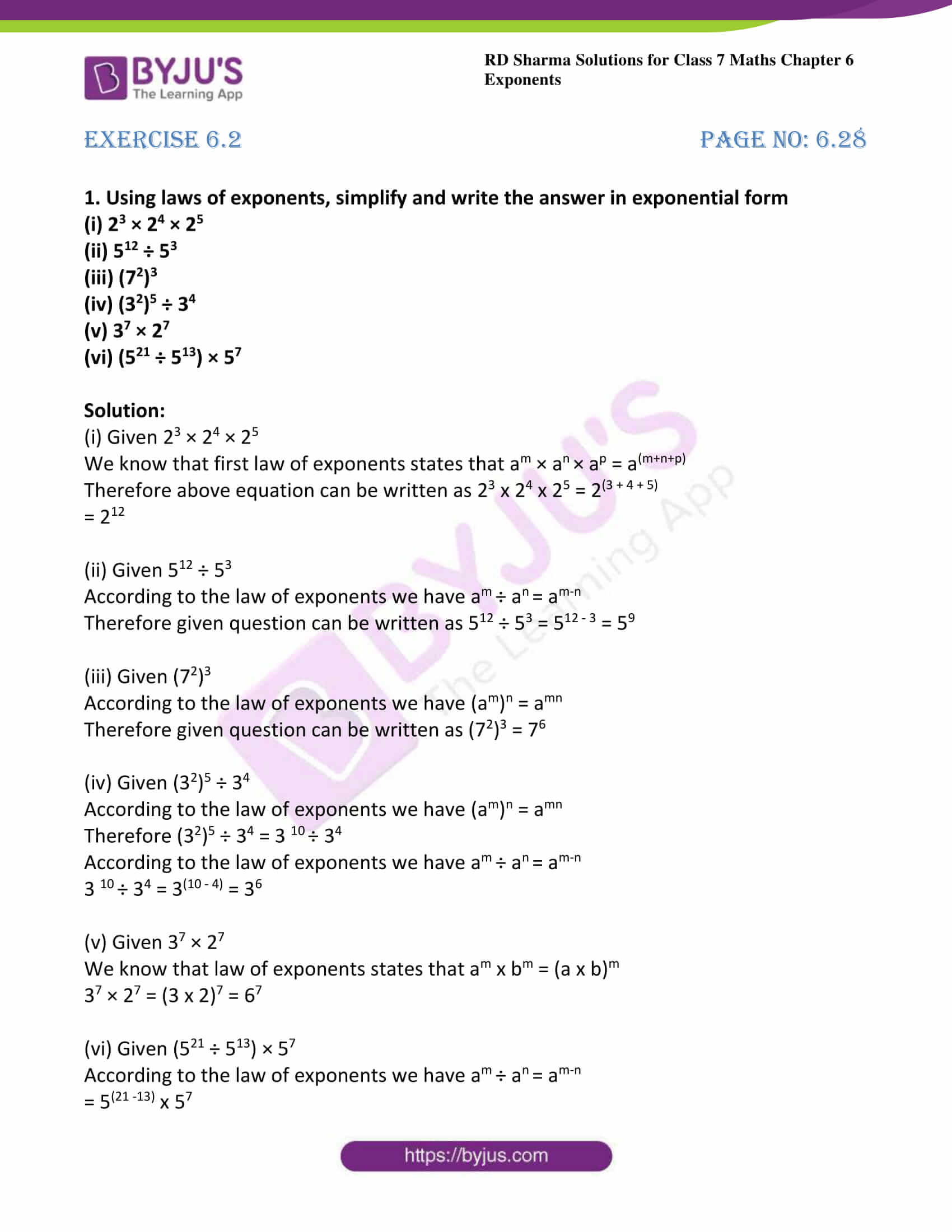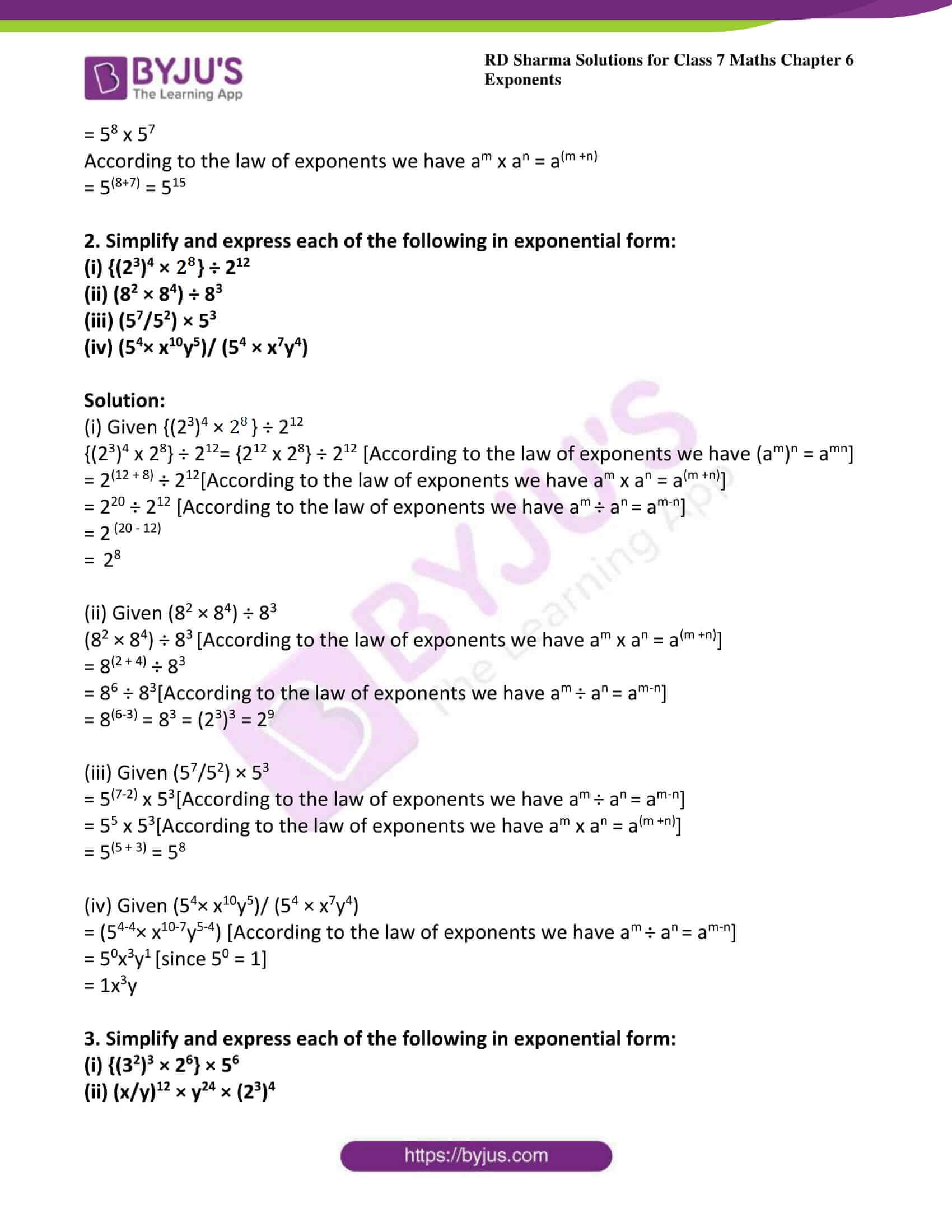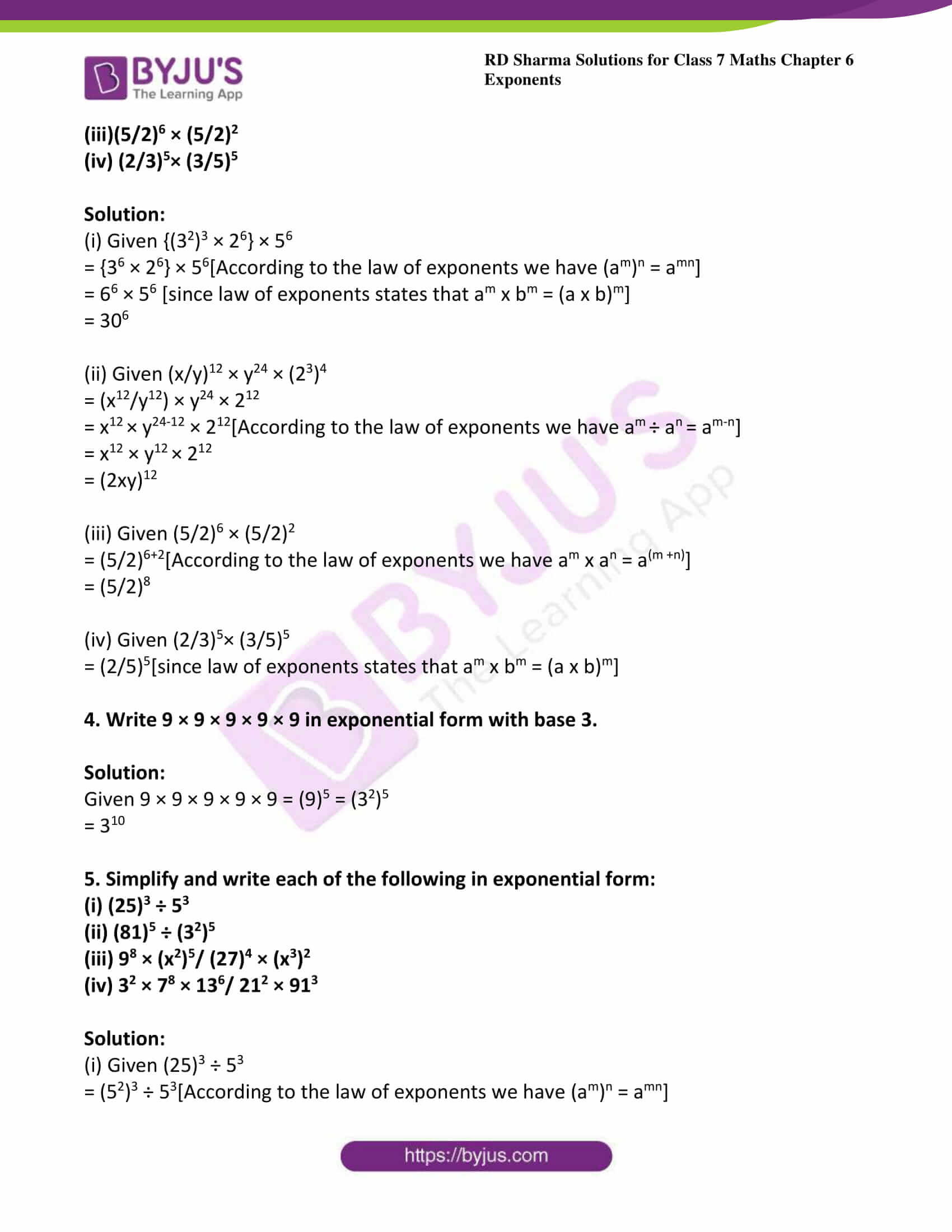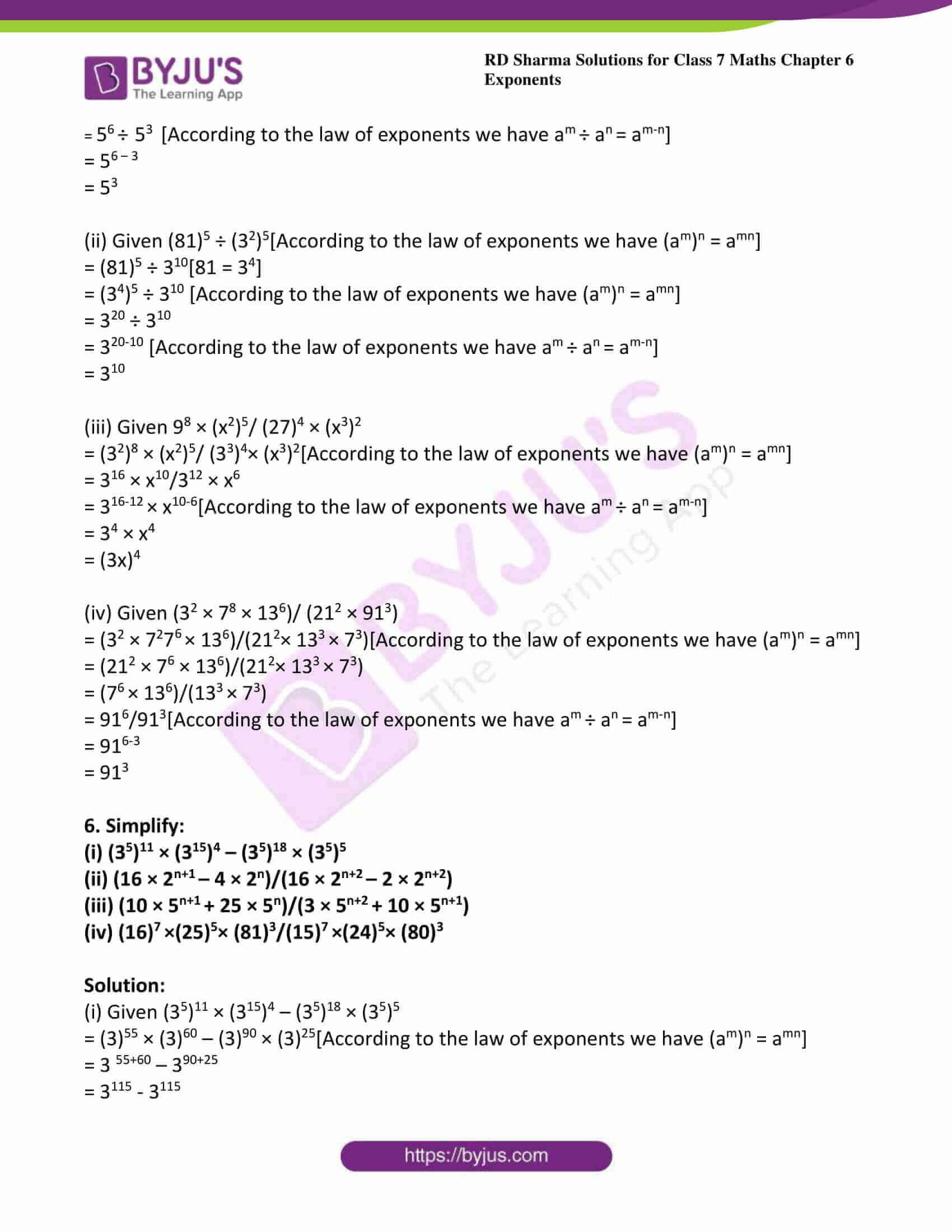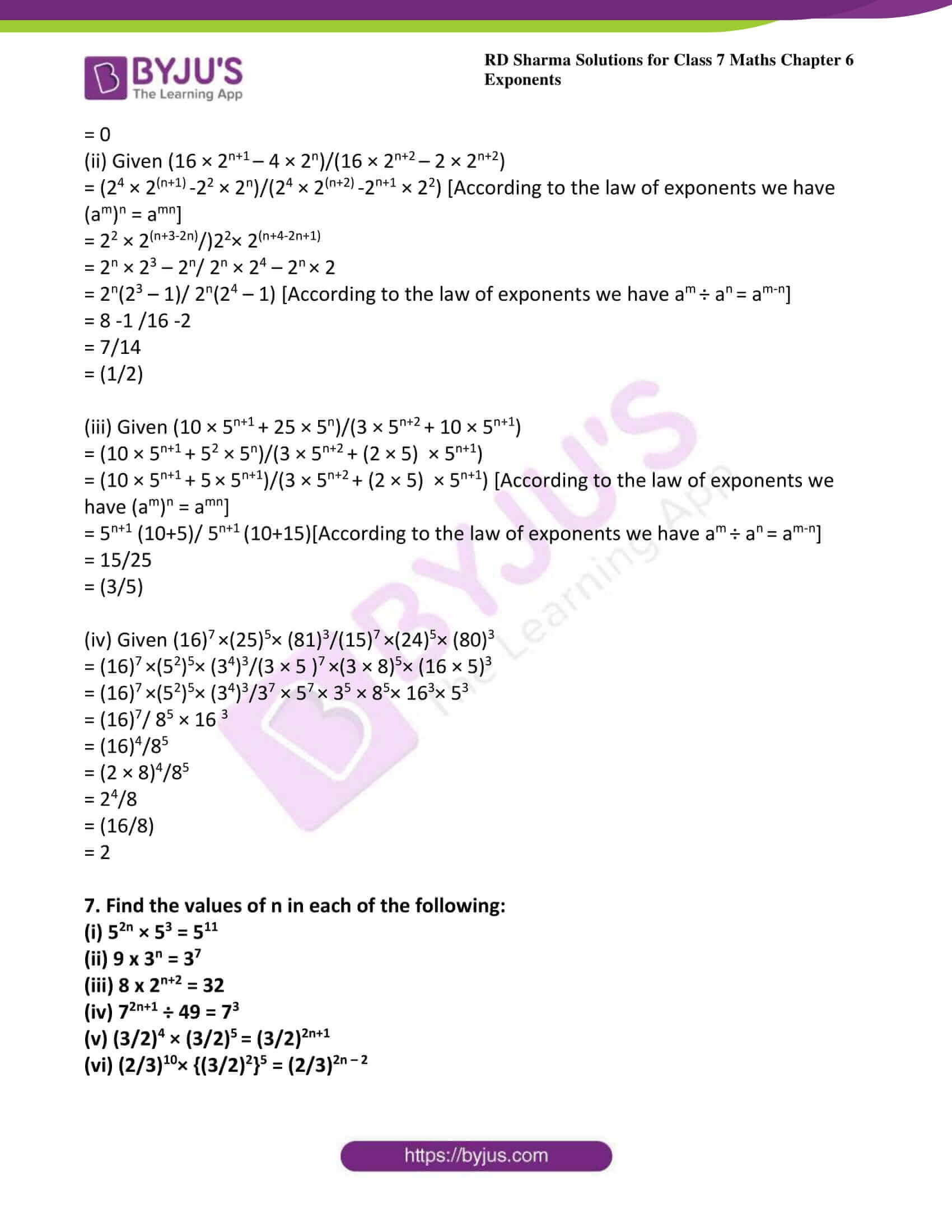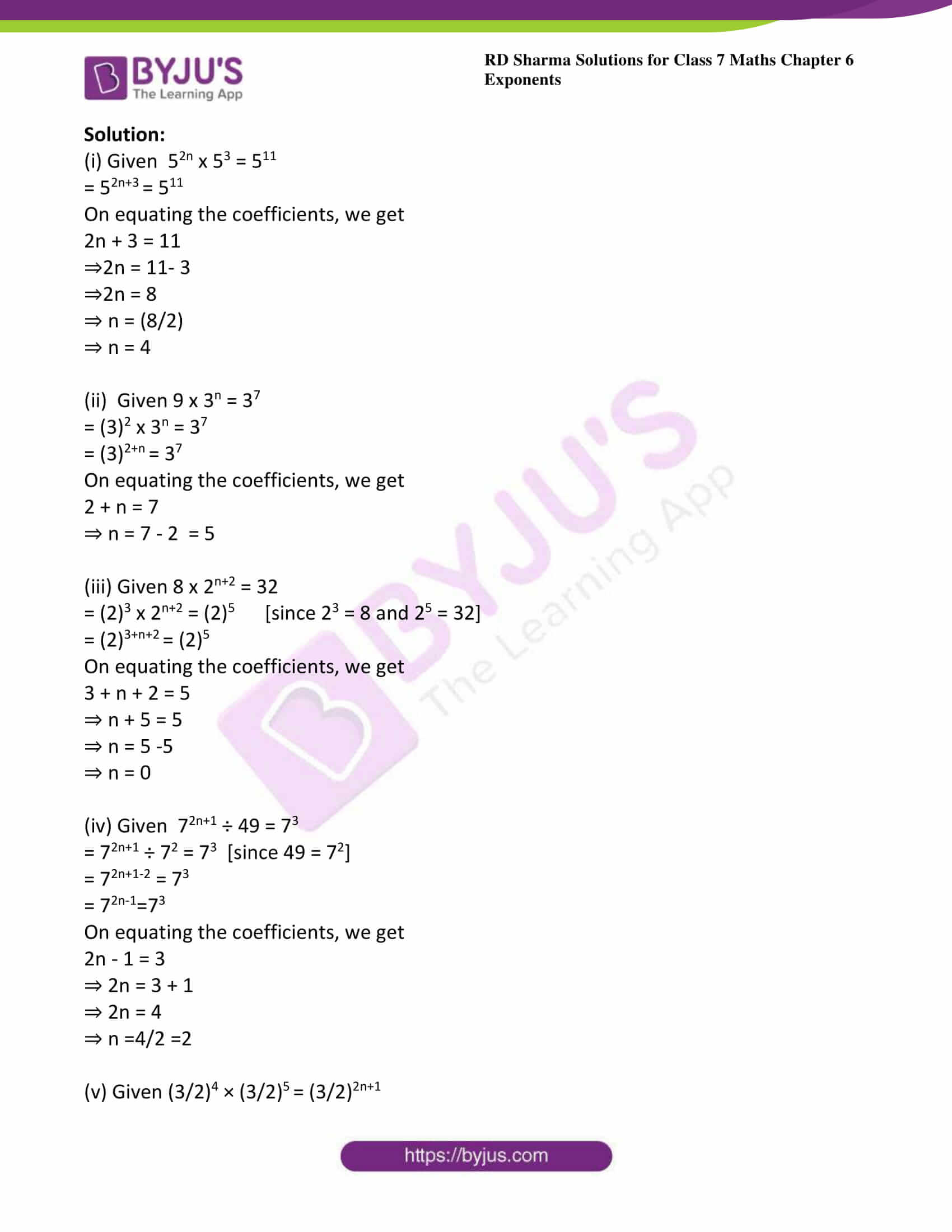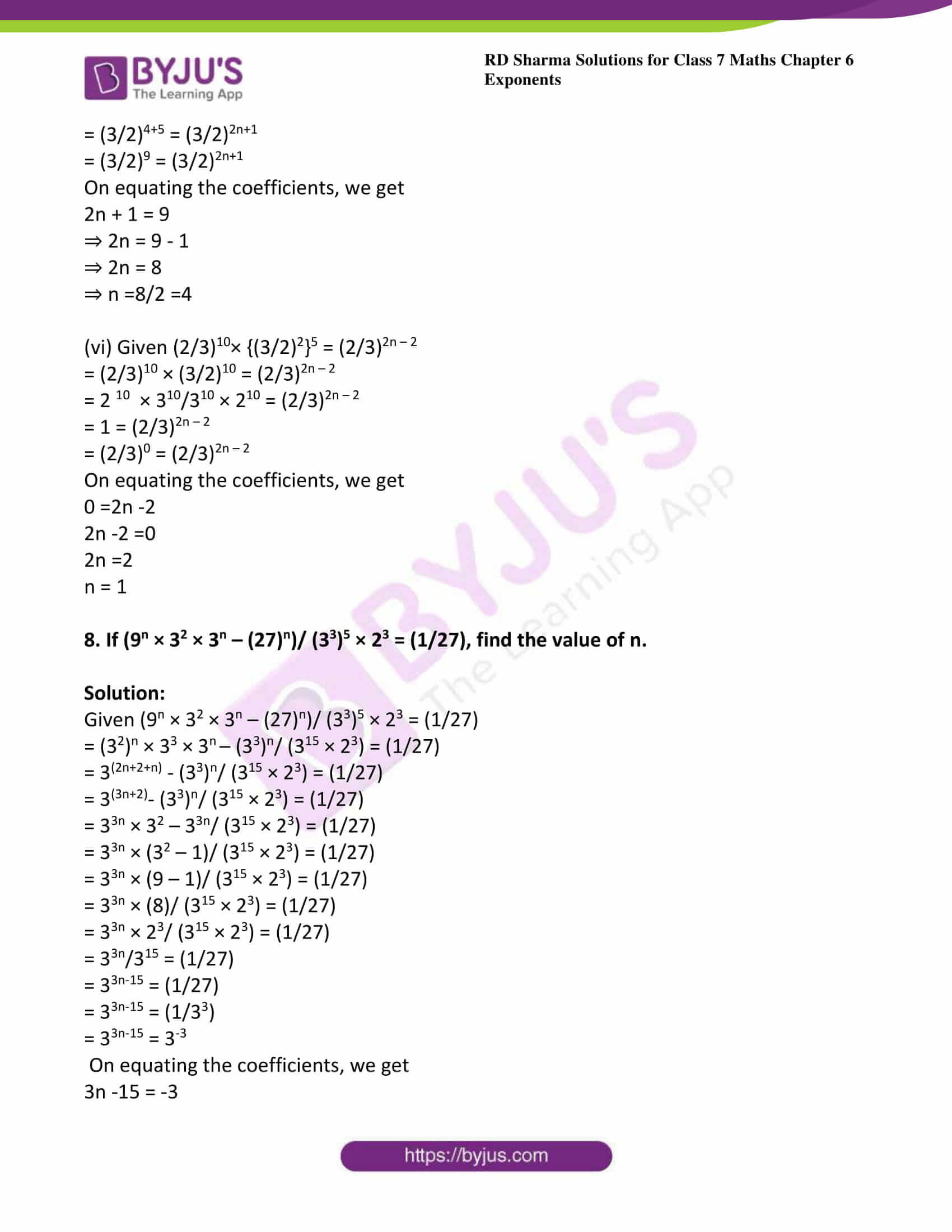### Access answers to Maths RD Sharma Solutions For Class 7 Chapter 6 – Exponents Exercise 6.2

1. Using laws of exponents, simplify and write the answer in exponential form

(i) 23 × 24 × 25

(ii) 512 ÷ 53

(iii) (72)3

(iv) (32)5 ÷ 34

(v) 37 × 27

(vi) (521 ÷ 513) × 57

Solution:

(i) Given 23 × 24 × 25

We know that first law of exponents states that am × a× ap = a(m+n+p)

Therefore above equation can be written as 23 x 24 x 25 = 2(3 + 4 + 5)

= 212

(ii) Given 512 ÷ 53

According to the law of exponents we have a÷ a= am-n

Therefore given question can be written as 512 ÷ 53 = 512 – 3 = 59

(iii) Given (72)3

According to the law of exponents we have (am)n = amn

Therefore given question can be written as (72)3 = 76

(iv) Given (32)5 ÷ 34

According to the law of exponents we have (am)n = amn

Therefore (32)5 ÷ 34 = 3 10 ÷ 34

According to the law of exponents we have a÷ a= am-n

3 10 ÷ 34 = 3(10 – 4) = 36

(v) Given 37 × 27

We know that law of exponents states that am x bm = (a x b)m

37 × 27 = (3 x 2)7 = 67

(vi) Given (521 ÷ 513) × 57

According to the law of exponents we have a÷ a= am-n

= 5(21 -13) x 57

= 58 x 57

According to the law of exponents we have am x an = a(m +n)

= 5(8+7) = 515

2. Simplify and express each of the following in exponential form:

(i) {(23)4 × 28} ÷ 212

(ii) (82 × 84) ÷ 83

(iii) (57/52) × 53

(iv) (54× x10y5)/ (54 × x7y4)

Solution:

(i) Given {(23)4 × 28} ÷ 212

{(23)4 x 28} ÷ 212= {212 x 28} ÷ 212 [According to the law of exponents we have (am)n = amn]

= 2(12 + 8) ÷ 212[According to the law of exponents we have am x an = a(m +n)]

= 220 ÷ 212 [According to the law of exponents we have a÷ a= am-n]

= 2 (20 – 12)

=  28

(ii) Given (82 × 84) ÷ 83

(82 × 84) ÷ 83 [According to the law of exponents we have am x an = a(m +n)]

= 8(2 + 4) ÷ 83

= 86 ÷ 83[According to the law of exponents we have a÷ a= am-n]

= 8(6-3) = 83 = (23)3 = 29

(iii) Given (57/52) × 53

= 5(7-2) x 53[According to the law of exponents we have a÷ a= am-n]

= 55 x 53[According to the law of exponents we have am x an = a(m +n)]

= 5(5 + 3) = 58

(iv) Given (54× x10y5)/ (54 × x7y4)

= (54-4× x10-7y5-4) [According to the law of exponents we have a÷ a= am-n]

= 50x3y1 [since 50 = 1]

= 1x3y

3. Simplify and express each of the following in exponential form:

(i) {(32)3 × 26} × 56

(ii) (x/y)12 × y24 × (23)4

(iii)(5/2)6 × (5/2)2

(iv) (2/3)5× (3/5)5

Solution:

(i) Given {(32)3 × 26} × 56

= {36 × 26} × 56[According to the law of exponents we have (am)n = amn]

= 66 × 56 [since law of exponents states that am x bm = (a x b)m]

= 306

(ii) Given (x/y)12 × y24 × (23)4

= (x12/y12) × y24 × 212

= x12 × y24-12 × 212[According to the law of exponents we have a÷ a= am-n]

= x12 × y12 × 212

= (2xy)12

(iii) Given (5/2)6 × (5/2)2

= (5/2)6+2[According to the law of exponents we have am x an = a(m +n)]

= (5/2)8

(iv) Given (2/3)5× (3/5)5

= (2/5)5[since law of exponents states that am x bm = (a x b)m]

4. Write 9 × 9 × 9 × 9 × 9 in exponential form with base 3.

Solution:

Given 9 × 9 × 9 × 9 × 9 = (9)5 = (32)5

= 310

5. Simplify and write each of the following in exponential form:

(i) (25)3 ÷ 53

(ii) (81)5 ÷ (32)5

(iii) 98 × (x2)5/ (27)4 × (x3)2

(iv) 32 × 78 × 136/ 212 × 913

Solution:

(i) Given (25)3 ÷ 53

= (52)3 ÷ 53[According to the law of exponents we have (am)n = amn]

= 56 ÷ 53 [According to the law of exponents we have a÷ a= am-n]

= 56 – 3

= 53

(ii) Given (81)5 ÷ (32)5[According to the law of exponents we have (am)n = amn]

= (81)5 ÷ 310[81 = 34]

= (34)5 ÷ 310 [According to the law of exponents we have (am)n = amn]

= 320 ÷ 310

= 320-10 [According to the law of exponents we have a÷ a= am-n]

= 310

(iii) Given 98 × (x2)5/ (27)4 × (x3)2

= (32)8 × (x2)5/ (33)4× (x3)2[According to the law of exponents we have (am)n = amn]

= 316 × x10/312 × x6

= 316-12 × x10-6[According to the law of exponents we have a÷ a= am-n]

= 34 × x4

= (3x)4

(iv) Given (32 × 78 × 136)/ (212 × 913)

= (32 × 727× 136)/(212× 133 × 73)[According to the law of exponents we have (am)n = amn]

= (212 × 76 × 136)/(212× 133 × 73)

= (76 × 136)/(133 × 73)

= 916/913[According to the law of exponents we have a÷ a= am-n]

= 916-3

= 913

6. Simplify:

(i) (35)11 × (315)4 – (35)18 × (35)5

(ii) (16 × 2n+1 – 4 × 2n)/(16 × 2n+2 – 2 × 2n+2)

(iii) (10 × 5n+1 + 25 × 5n)/(3 × 5n+2 + 10 × 5n+1)

(iv) (16)7 ×(25)5× (81)3/(15)7 ×(24)5× (80)3

Solution:

(i) Given (35)11 × (315)4 – (35)18 × (35)5

= (3)55 × (3)60 – (3)90 × (3)25[According to the law of exponents we have (am)n = amn]

= 3 55+60 – 390+25

= 3115 – 3115

= 0

(ii) Given (16 × 2n+1 – 4 × 2n)/(16 × 2n+2 – 2 × 2n+2)

= (24 × 2(n+1) -22 × 2n)/(24 × 2(n+2) -2n+1 × 22) [According to the law of exponents we have (am)n = amn]

= 22 × 2(n+3-2n)/)22× 2(n+4-2n+1)

= 2n × 23 – 2n/ 2n × 24 – 2n × 2

= 2n(23 – 1)/ 2n(24 – 1) [According to the law of exponents we have a÷ a= am-n]

= 8 -1 /16 -2

= 7/14

= (1/2)

(iii) Given (10 × 5n+1 + 25 × 5n)/(3 × 5n+2 + 10 × 5n+1)

= (10 × 5n+1 + 52 × 5n)/(3 × 5n+2 + (2 × 5) × 5n+1)

= (10 × 5n+1 + 5 × 5n+1)/(3 × 5n+2 + (2 × 5) × 5n+1) [According to the law of exponents we have (am)n = amn]

= 5n+1 (10+5)/ 5n+1 (10+15)[According to the law of exponents we have a÷ a= am-n]

= 15/25

= (3/5)

(iv) Given (16)7 ×(25)5× (81)3/(15)7 ×(24)5× (80)3

= (16)7 ×(52)5× (34)3/(3 × 5 )7 ×(3 × 8)5× (16 × 5)3

= (16)7 ×(52)5× (34)3/37 × 57 × 35 × 85× 163× 53

= (16)7/ 85 × 16 3

= (16)4/85

= (2 × 8)4/85

= 24/8

= (16/8)

= 2

7. Find the values of n in each of the following:

(i) 52n × 53 = 511

(ii) 9 x 3n = 37

(iii) 8 x 2n+2 = 32

(iv) 72n+1 ÷ 49 = 73

(v) (3/2)4 × (3/2)5 = (3/2)2n+1

(vi) (2/3)10× {(3/2)2}5 = (2/3)2n – 2

Solution:

(i) Given 52n x 53 = 511

= 52n+3 = 511

On equating the coefficients, we get

2n + 3 = 11

⇒2n = 11- 3

⇒2n = 8

⇒ n = (8/2)

⇒ n = 4

(ii)  Given 9 x 3n = 37

= (3)2 x 3n = 37

= (3)2+n = 37

On equating the coefficients, we get

2 + n = 7

⇒ n = 7 – 2  = 5

(iii) Given 8 x 2n+2 = 32

= (2)3 x 2n+2 = (2)5      [since 23 = 8 and 25 = 32]

= (2)3+n+2 = (2)5

On equating the coefficients, we get

3 + n + 2 = 5

⇒ n + 5 = 5

⇒ n = 5 -5

⇒ n = 0

(iv) Given  72n+1 ÷ 49 = 73

= 72n+1 ÷ 72 = 73  [since 49 = 72]

= 72n+1-2 = 73

= 72n-1=73

On equating the coefficients, we get

2n – 1 = 3

⇒ 2n = 3 + 1

⇒ 2n = 4

⇒ n =4/2 =2

(v) Given (3/2)4 × (3/2)5 = (3/2)2n+1

= (3/2)4+5 = (3/2)2n+1

= (3/2)9 = (3/2)2n+1

On equating the coefficients, we get

2n + 1 = 9

⇒ 2n = 9 – 1

⇒ 2n = 8

⇒ n =8/2 =4

(vi) Given (2/3)10× {(3/2)2}5 = (2/3)2n – 2

= (2/3)10 × (3/2)10 = (2/3)2n – 2

= 2 10 × 310/310 × 210 = (2/3)2n – 2

= 1 = (2/3)2n – 2

= (2/3)0 = (2/3)2n – 2

On equating the coefficients, we get

0 =2n -2

2n -2 =0

2n =2

n = 1

8. If (9n × 32 × 3n – (27)n)/ (33)5 × 23 = (1/27), find the value of n.

Solution:

Given (9n × 32 × 3n – (27)n)/ (33)5 × 23 = (1/27)

= (32)n × 33 × 3n – (33)n/ (315 × 23) = (1/27)

= 3(2n+2+n) – (33)n/ (315 × 23) = (1/27)

= 3(3n+2)– (33)n/ (315 × 23) = (1/27)

= 33n × 32 – 33n/ (315 × 23) = (1/27)

= 33n × (32 – 1)/ (315 × 23) = (1/27)

= 33n × (9 – 1)/ (315 × 23) = (1/27)

= 33n × (8)/ (315 × 23) = (1/27)

= 33n × 23/ (315 × 23) = (1/27)

= 33n/315 = (1/27)

= 33n-15 = (1/27)

= 33n-15 = (1/33)

= 33n-15 = 3-3

On equating the coefficients, we get

3n -15 = -3

⇒ 3n = -3 + 15

⇒ 3n = 12

⇒ n = 12/3 = 4